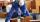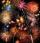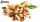# One third

If 3/5 is 360, how much is 1/3?

Result

y =  200

#### Solution:Leave us a comment of example and its solution (i.e. if it is still somewhat unclear...):

Showing 0 comments:Be the first to comment!#### To solve this example are needed these knowledge from mathematics:

Need help calculate sum, simplify or multiply fractions? Try our fraction calculator. Do you have a linear equation or system of equations and looking for its solution? Or do you have quadratic equation?

## Next similar examples:

1. Unknown numberI think the number - its sixth is 3 smaller than its third.
2. NumberI think the number. If I add to its third seven I get same as when to its quarter add 8. Which is the number?
3. ClassroomOne-eighth of 9th class was interested in studying at a grammar school, at a business academy one sixth, at secondary vocational schools quarter, to SOU one third and the remaining three students were interested in the school of art direction. How many st
4. The bunsKate, Zofia and Peter Liked buns. Even today, their grandmother prepare their favorite meal. Katka eats 4 bunches, Žofia 3 and Petra eats 5 buns. Their grandmother said to them, "My inmate will you know how many buns I have been make today, if those you ea
5. EqnSolve equation with fractions: 2x/3-50=40+x/4
6. PearsThere were pears in the basket, I took two-fifths of them, and left six in the basket. How many pears did I take?
7. EquationFind x: x + 1/2 = 1/3
8. Missing numberBlank +1/6 =3/2 find the missing number
9. TreesFrom the total number of trees in the orchard, there are two-fifths pearls and apples are three eighty. The rest of the trees are 9 ceremonial. How many trees are in the set?
10. Bricklayers8 bricklayers build a house for 630 days. How many bricklayers have to add after 150 days, so then the whole building completed in (next) 320 days?
11. UN 1If we add to an unknown number his quarter, we get 210. Identify unknown number.
12. King's birthdayTo celebrate the king's birthday workers fire 1/5 all purchased rockets. To celebrate the Queen's birthday fire 1/6 of the remaining rockets and to celebrate the birthday of king's son remaining 15,000 rockets. How many rockets they purchased?
13. Trees3/5 trees are apples, cherries are 1/3. 5 trees are pear. How many is the total number of trees?
14. Bag of peanutsJoe eat 1/3 of a bag of peanuts, mark eat 1/4 of the remaining in the bag of peanuts, Alvin eat 1/2 of the remaining bag of peanuts, peter eat 10 peanuts, there are 71 peanuts left. Hon many peanuts were in the bags?
15. EquationSolve equation and check the result: 1.4x - 3/2 + x - 9,8 = x + 0,4/3 - 7 + 1,6/6
16. Unknown number 6Determine the unknown number, which is by 1.5 greater than its fourth.
17. Simple equationSolve for x: 3(x + 2) = x - 18